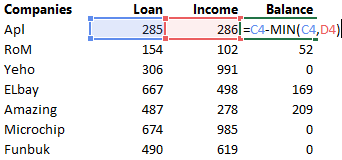Take a look at this case and think about how are you going to solve it without using IF / AND or any other Logical function

Case 1Case 2DOWNLOAD THE CASES HERE – and give it a shot solving it without using IF/AND or any logical function. Do not scroll down till you give it a reasonably good shot at solving them 😀

## The Solution

The smart and optimized way to solve this is to use the MIN function for Case 1All I am doing is

1. Finding the MIN of Loan and Income – because that is what we are paying off
2. Subtracting that from Loan amount to get the balance

Here is how you can solve the 2nd case using the MAX Function1. (D17- C17)*\$E\$15 is going to give me the tax payable (Profit x Tax Rate)
2. I then use the MAX Function to limit tax payable to 0 (zero) if it is a negative number

Smart huh? 😎

## Where can you replace the IF function with MIN or MAX Logic?

Wherever you want the smaller or the greater of the 2 numbers (or even a formula) try using the MIN or MAX function rather than writing a long IF statement.

The result will be = smarter logic and smaller formula

## Have you used MIN MAX Logic before?

Please let me know if you have been using the IF function which can now be changed to a smarter MIN MAX logic or if you already are pro at this?